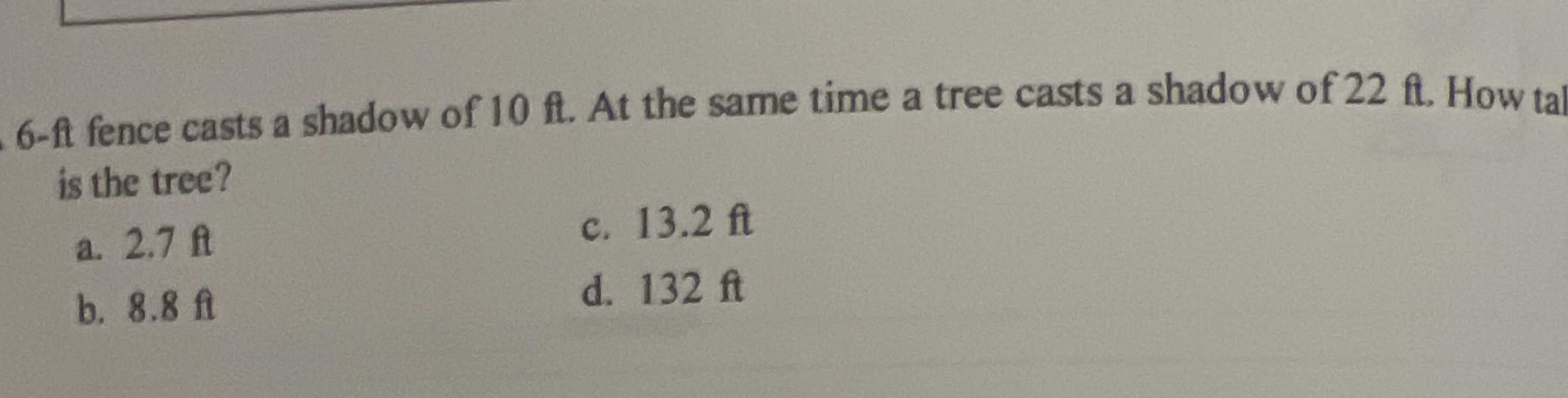### Still have math questions?

Trigonometry
Question$$6$$ - ft fence casts a shadow of $$10 ft$$ . At the same time a tree casts a shadow of $$22 ft$$ . How tal is the tree? a. $$2.7 ft$$ c. $$13.2 ft$$

b. $$8.8 ft$$ d. $$132 ft$$

tan x = 6/10 = 0.6

also

tan x = h/22

h/22 = 0.6

h = 22 x 0.6

h = 13.2 ft

Solution
View full explanation on CameraMath App.